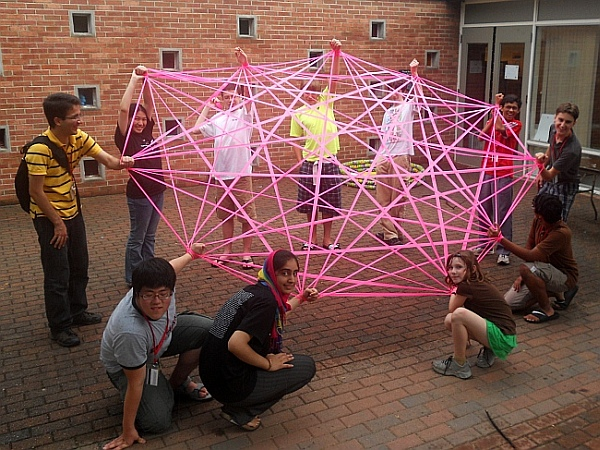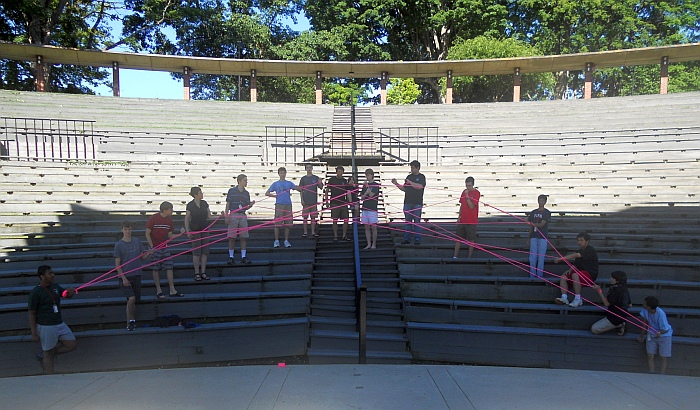Math Monday: Human-Scale String Constructions
SEPTEMBER 20, 2010

by George Hart

A complete graph is what mathematicians call a collection of items in which every pair is connected. If the items are spaced evenly around a circle and the connections are shown as straight lines, the lines form an attractive pattern of concentric circles.This is a complete graph with eleven vertices. We made it at Mathcamp 2010 using plastic surveyor’s tape. There is a simple algorithm for constructing it in which people stand in a circle, and pass the roll from one to the next while counting aloud, wrapping it around their left wrist at the proper intervals.A similar algorithm, executed by people standing in two straight lines, gives this large parabola. The construction steps for both of these figures can be seen in the additional photos here.

The “envelope of the lines” is a parabola. I am assuming people stand at integer points on the axes and there is a segment from (x,0) to (0,y), where x+y=c. (c is a constant, roughly half the number of people available.) For example, with c=10, there could be a segment from (5,0) to (0,5), and one from (6,0) to (0,4), and generally from (n,0) to (0, 10-n). For very large or small n, these tangent lines approach a 45 degree slope, so they become parallel, which is characteristic of a parabola.